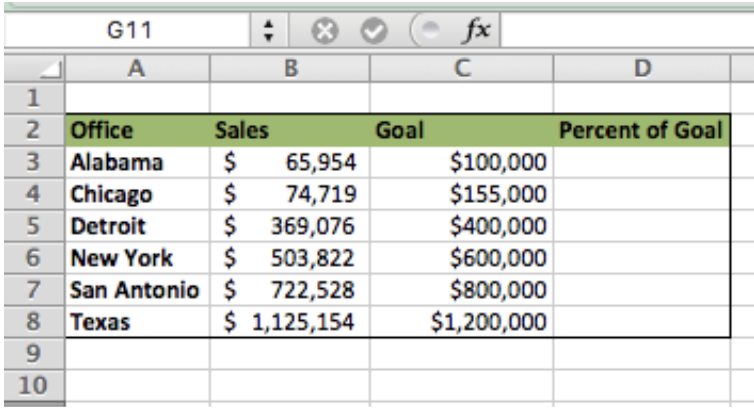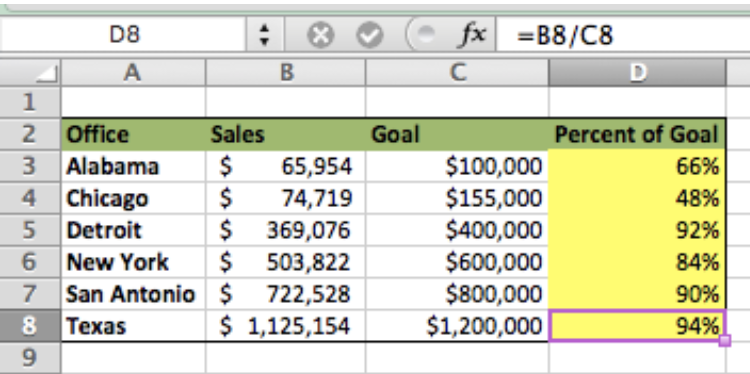Get instant live expert help with Excel or Google Sheets“My Excelchat expert helped me in less than 20 minutes, saving me what would have been 5 hours of work!”

#### Post your problem and you'll get expert help in seconds

Your message must be at least 40 characters
Our professional experts are available now. Your privacy is guaranteed.

# How to Calculate Percent of Goal

Quickly find the Percent of Goal using simple Excel formulas. This tutorial will step you through how to calculate the percentage completion of any numeric goal..

## Calculation:

`Actual Results / Goal = Percent of Goal`

## Setting up Our Data

• Setup our data so that we have the Actual Data in Column B, the Goal in Column C and a blank Column D which will be where we enter the formulaFigure 1 – Setting up the Data

Calculate Percent of Goal

• In cell D3 type this formulas `=B3/C3`
• Copy the formula in D3 down to D8
• We see in the example Excel calculates the percent goal for each office
• Excel defaults the result in decimal format, so be sure to change the cell format to percentFigure 2 – Calculating the Percent of Goal

*This formula will not work with a negative goal. Instead, use this formula:

`= (actual-goal)/ABS(goal)+1`

## Instant Connection to an Expert through our Excelchat Service:

Most of the time, the problem you will need to solve will be more complex than a simple application of a formula or function. If you want to save hours of research and frustration, try our live Excelchat service! Our Excel Experts are available 24/7 to answer any Excel question you may have. We guarantee a connection within 30 seconds and a customized solution within 20 minutes.

### Did this post not answer your question? Get a solution from connecting with the expert.Another blog reader asked this question today on Excelchat:## Subscribe to Excelchat.coAnother blog reader asked this question today on Excelchat: